Next: About this document ... Up: lab_template Previous: lab_template

Subsections

# The Definite Integral

## Purpose

The purpose of this lab is to introduce you to Maple commands for computing definite and indefinite integrals.

## Background

### Introyduction

There are two main ways to think of the definite integral. The easiest one to understand is as a means for computing areas (and volumes). The second way the definite integral is used is as a sum. That is, we use the definite integral to add things up''. Here are some examples.
• Computing net or total distance traveled by a moving object.
• Computing average values, e.g. centroids and centers of mass, moments of inertia, and averages of probability distributions.
This lab is intended to introuduce you to Maple commands for computing integrals, including applications of integrals.

### Definite and indefinite integrals with Maple

The basic Maple command for computing definite and indefinite integrals is the int command. Suppose you wanted to compute the following definite integral with Maple.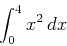The command to use is shown below.

> int(x^2,x=0..4);


Notice that Maple gives an exact answer, as a fraction. If you want a decimal approximation to an integral, you just put an evalf command around the int command, as shown below.

> evalf(int(x^2,x=0..4));


To compute an indefinite integral with Maple, you just leave out the range for the limits of integration, as shown below.

> int(x^2,x);

Note that Maple does not include a constant of integration.

You can also use the Maple int command with functions or expressions you have defined in Maple. For example, suppose you wanted to find area under the curve of the functionon the interval. Then you can define this function in Maple with the command

> f := x -> x*sin(x);

and then use this definition as shown below.
> int(f(x),x=0..Pi);


You can also simply give the expression corresponding to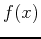a label in Maple, and then use that label in subsequent commands as shown below. However, notice the difference between the two methods. You are urged to choose one or the other, so you don't mix the syntax up.

> p := x*sin(x);
> int(p,x=0..Pi);

If you want to find the area bounded by the graph of two functions, you should first plot both functions on the same graph. You can then find the intersection points using either the solve or fsolve command. Once this is done, you can calculate the definite integral in Maple. An example below illustrates how this can be done in Maple by finding the area bounded by the graphs ofand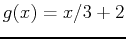:
> f := x-> -x^2+4*x+6;
> g := x-> x/3+2;
> plot({f(x),g(x)},x=-2..6);
> a := fsolve(f(x)=g(x),x=-2..0);
> b := fsolve(f(x)=g(x),x=4..6);
> int(f(x)-g(x),x=a..b);


### Average value of a function

If a function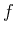is integrable over a closed interval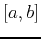, then the average value of, denoted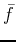, on this interval is:Note that the average value is just a number. For example, suppose you wanted to compute the average value of the functionover the interval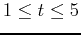. The following Maple command would do the job.
> int(-16*t^2+100*t,t=1..5)/(5-1);


## Exercises

1. Use the function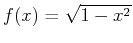and a definite integral to show that the area of a circle of radius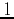is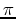.
2. Find the area of the region bounded by the curves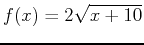and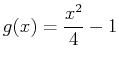.
3. Find the area of the region bounded by the curves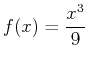and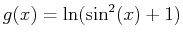.
4. Use a definite integral to find the area of the triangle with vertices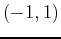,and.
5. Find the average velocity of a particle moving in one dimension with velocity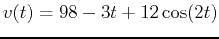given in feet per second from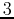to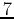seconds.Next: About this document ... Up: lab_template Previous: lab_template
Dina J. Solitro-Rassias
2012-09-20